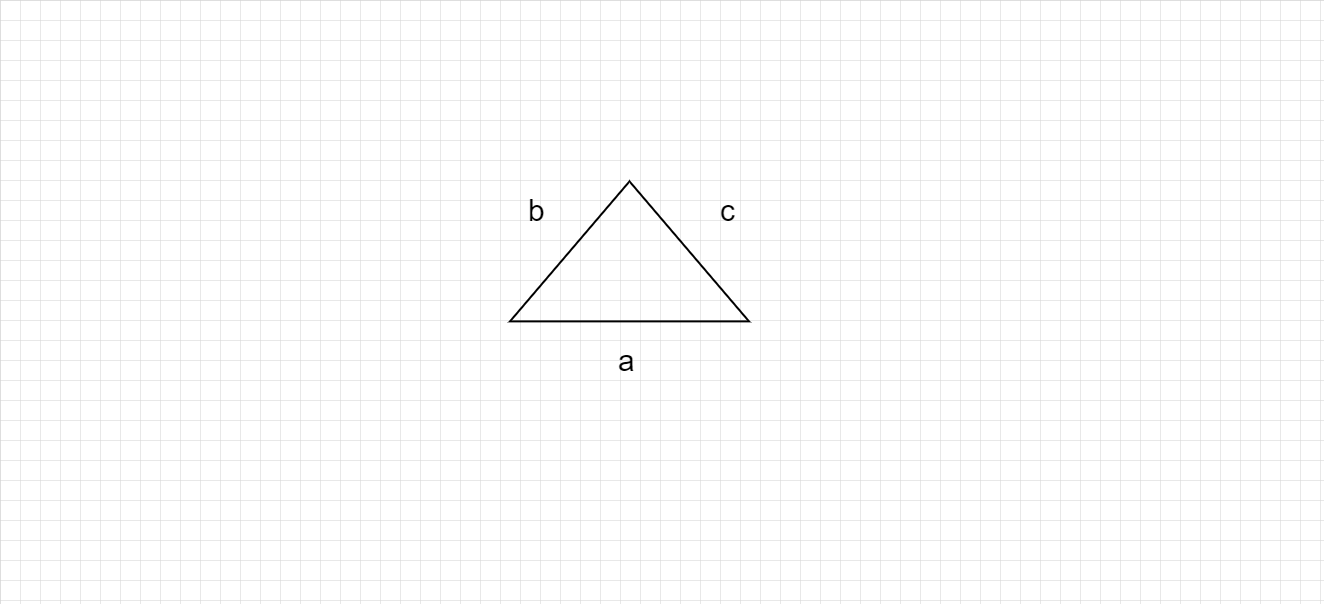# What is perimeter and how to do it ?

Perimeter :

In geometry, perimeter can be defined as the path or the boundary that surrounds a shape. It can also be defined as the length of the outline of a shape.

Perimeter of triangle :

Perimeter of the triangle below with sides of length a units, b units and c units $= (a + b + c)$ units.Similarly, to find the perimeter of a complex figure, add the lengths of all the sides of the figure.

Updated on: 10-Oct-2022

42 Views